Courses
Courses for Kids
Free study material
Offline Centres
More

# NCERT Solutions for Class 9 Maths Chapter 11 - ExerciseLast updated date: 29th Nov 2023
Total views: 532.8k
Views today: 5.32k

## Access NCERT Solutions For Class 9 Mathematics Chapter 11- Constructions

Exercise 11.2

1. Construct a triangle $\mathbf{ABC}$ in which $\mathbf{BC}=\mathbf{7}$ cm, $\angle \mathbf{B}=\mathbf{7}{{\mathbf{5}}^{\mathbf{o}}}$ and $\mathbf{AB}+\mathbf{AC}=\mathbf{13}$ cm.

Ans: To draw the triangle $ABC$, follow the procedure listed below.

• First, draw a $7$ cm long line segment $BC$.

• Then, construct a ${{75}^{\circ }}$ angle $\angle XBC$ at $B$.

• After that, from the line $BX$, cut a line segment $BD$ of length $13$ cm (which is equivalent with $AB+AC$).

• Next connect $DC$ and construct an angle $\angle DCY$ equivalent to $\angle BDC$, by measuring the same arc radius as the angle $\angle BDC$ have.

• Finally, suppose that the line $CY$ meet $BX$ at the point $A$.

• Thus, $\Delta ABC$ forms the triangle needed.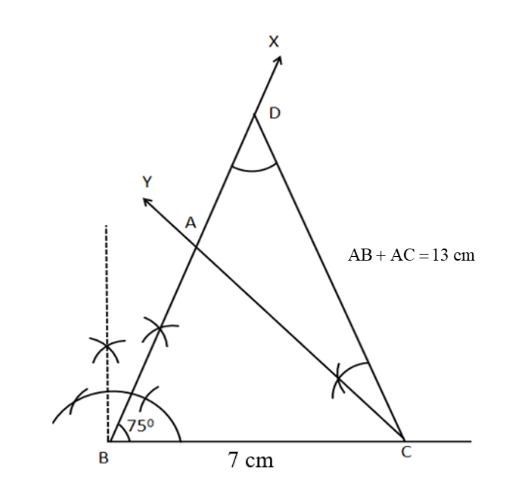2. Construct a triangle $\mathbf{ABC}$ in which $\mathbf{BC}=\mathbf{8}$ cm, $\angle \mathbf{B}=\mathbf{4}{{\mathbf{5}}^{\mathbf{o}}}$, and $\mathbf{AB}-\mathbf{AC}=\mathbf{3}.\mathbf{5}$ cm.

Ans: To construct the triangle $ABC$, follow the procedure listed below.

• First, draw a $8$ cm long line-segment $BC$.

• Then, construct a ${{45}^{\circ }}$ angle $\angle XBC$ at $B$.

• Next, from the line $BX$, split a line segment $BD=3.5$cm (which is equivalent with $AB-AC$).

• After that, connect $DC$ and construct $PQ$ as the perpendicular bisector $PQ$ of the line segment $DC$.

• Finally, suppose that the line $BX$ meet $PQ$ at the point $A$ and connect $AC$.

• Hence, $\Delta ABC$ forms the triangle needed.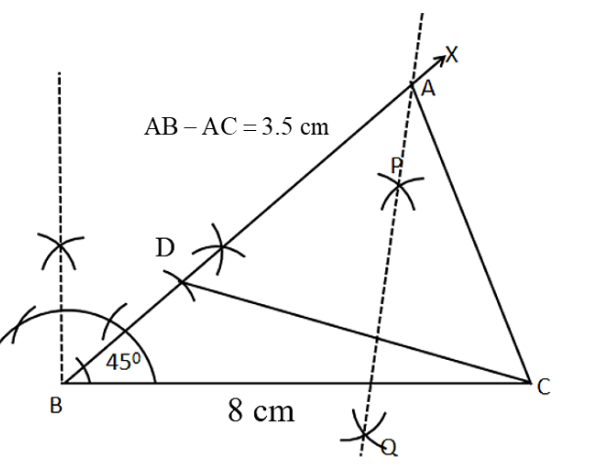3. Construct a triangle $\mathbf{PQR}$ in which $\mathbf{QR}=\mathbf{6}$ cm, $\angle \mathbf{Q}=\mathbf{6}{{\mathbf{0}}^{\mathbf{o}}}$, and $\mathbf{PR}-\mathbf{PQ}=\mathbf{2}$ cm.

Ans: To construct the triangle $ABC$, follow the procedure listed below.

• First, draw a $6$ cm long line-segment $QR$.

• Then, construct a ${{60}^{\circ }}$ angle $\angle XQR$ at $Q$.

• After that, from the line segment $QT$ on the opposite side of the line segment $XQ$, split a $2$ cm line segment $QS$. (Since $PR>PQ$ and $PR-PQ=2$ cm.). Then connect $SR$.

• Next, construct $AB$ as the perpendicular bisector on the line segment $SR$.

• Finally, suppose that $AB$ meet $QX$ at $P$ and connect $PQ$ and $PR$.

• Hence, $\Delta \,PQR$ forms the triangle as needed.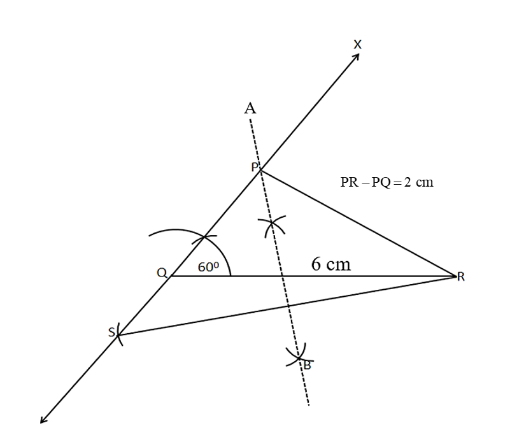4. Construct a triangle $\mathbf{XYZ}$ in which $\angle \mathbf{Y}=\mathbf{3}{{\mathbf{0}}^{\mathbf{o}}}$, $\angle \mathbf{Z}=\mathbf{9}{{\mathbf{0}}^{\mathbf{o}}}$, and $\mathbf{XY}+\mathbf{YZ}+\mathbf{ZX}=\mathbf{11}$ cm.

Ans: To construct the triangle $XYZ$, follow the procedure listed below.

• First, draw a line $AB$ of length $11$ cm. (Since, $XY+YZ+ZX=11$cm)

• Then, draw a ${{30}^{\circ }}$ angle $\angle PAB$ at the point $A$ and a ${{90}^{\circ }}$ angle QBA at $B$.

• After that, construct the bisectors of the angles $\angle PAB$ and $\angle QBA$ so that they meet together at $X$.

• Next, construct $ST$ and $UV$ as the perpendicular bisectors on the lines $AX$ and $BX$ respectively.

• Then, suppose that $ST$ and $UV$ meet the line $AB$ at the points $Y$ and $Z$ respectively.

• Finally, connecting $XY$ and $YZ$, $\Delta XYZ$ forms the triangle, we needed.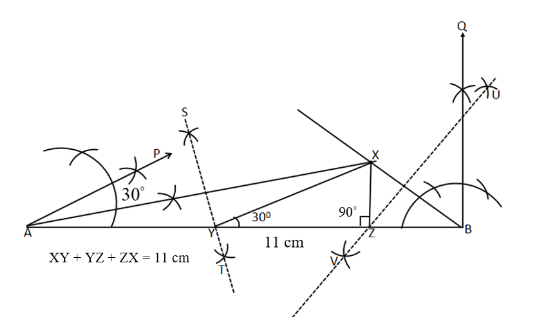5. Construct a right triangle whose base is $\mathbf{12}$ cm and sum of its hypotenuse and other side is $\mathbf{18}$ cm.

Ans: To construct the required right-triangle, follow the procedure listed below.

• First, draw a line segment $AB$ of length $12$ cm.

• Then, construct a ${{90}^{\circ }}$ angle $\angle XAB$ at the point $A$.

• After that, from the line $AX$, snip a line segment $AD$ of length $18$ cm (because the sum of the length of other two sides is $18$ cm). .

• Next, connect $DB$ and construct an angle $\angle DBY$ equivalent to $\angle ADB$.

• Suppose that, $BY$ meet the ray $AX$ at the point $C$.

• Finally, connect $AC$ and $BC$.

• Thus, $\Delta ABC$ forms the triangle, which we needed.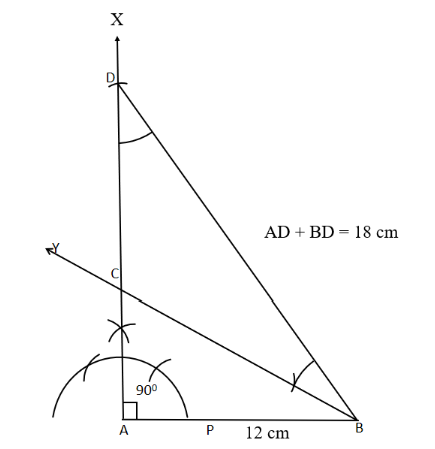## NCERT Solutions for Class 9 Maths Chapter 11 Constructions Exercise 11.2

Important Topics Covered in Exercise 11.2 of Class 9 Maths NCERT Solutions

Exercise 11.2 of Class 9 Maths NCERT Solutions is mainly focused on some constructions of triangles using their properties discussed in Chapter 7 and in previous classes. All the questions given in this exercise are based on the construction of triangles. The NCERT Solutions provided for this exercise will help students understand the concepts of triangles better.

Students are advised to practise all the questions provided in these solutions so that they can master these topics and they will be able to score high marks in exams. Students can download the solutions available in PDF format from this page for free.

Opting for the NCERT solutions for Ex 11.2 Class 9 Maths is considered as the best option for the CBSE students when it comes to exam preparation. This chapter consists of many exercises. Out of which we have provided the Exercise 11.2 Class 9 Maths NCERT solutions on this page in PDF format. You can download this solution as per your convenience or you can study it directly from our website/ app online.

Vedantu in-house subject matter experts have solved the problems/ questions from the exercise with the utmost care and by following all the guidelines by CBSE. Class 9 students who are thorough with all the concepts from the Subject Constructions textbook and quite well-versed with all the problems from the exercises given in it, then any student can easily score the highest possible marks in the final exam. With the help of this Class 9 Maths Chapter 11 Exercise 11.2 solutions, students can easily understand the pattern of questions that can be asked in the exam from this chapter and also learn the marks weightage of the chapter. So that they can prepare themselves accordingly for the final exam.

Besides these NCERT solutions for Class 9 Maths Chapter 11 Exercise 11.2, there are plenty of exercises in this chapter which contain innumerable questions as well. All these questions are solved/answered by our in-house subject experts as mentioned earlier. Hence all of these are bound to be of superior quality and anyone can refer to these during the time of exam preparation. In order to score the best possible marks in the class, it is really important to understand all the concepts of the textbooks and solve the problems from the exercises given next to it.

Do not delay any more. Download the NCERT solutions for Class 9 Maths Chapter 11 Exercise 11.2 from Vedantu website now for better exam preparation. If you have the Vedantu app in your phone, you can download the same through the app as well. The best part of these solutions is these can be accessed both online and offline as well.

### NCERT Solution Class 9 Maths of Chapter 11 All Exercises

 Chapter 11 - Constructions Exercises in PDF Format Exercise 11.1 5 Questions & Solutions (2 Short Answers, 2 Long Answer, 1 Very Long Answer)

NCERT Solutions for Class 9 Maths All Chapters

## FAQs on NCERT Solutions for Class 9 Maths Chapter 11 - Exercise

1. What topics and subtopics are covered in NCERT Solutions for Class 9 Maths Chapter 11 Constructions (Ex 11.2) Exercise 11.2 provided by Vedantu?

The introduction of constructions, the construction of bisectors, perpendicular bisectors, angles, and triangles are the primary topics addressed in NCERT Solutions for Class 9 Maths Chapter 11 Constructions (Ex 11.2) Exercise 11.2. The concept of geometrical shapes like triangles and how they are constructed is the basis for the problems that make up Constructions Exercise 11.2. From the viewpoint of an exam, these exercise questions are essential since they enable students to recognize and resolve the range of questions that might be asked about the subject. Additionally, NCERT Solutions for Class 9 Maths Chapter 11 Constructions (Ex 11.2) Exercise 11.2 provided by Vedantu helps students in understanding the significance and practical applications of a given subject.

2. What does it mean to Bisect a segment and how can you tell if a Line is a Perpendicular Bisector?

A bisector is a line that divides anything into two equal portions. To divide a line segment into two equal sections is to "bisect" it. A line segment that cuts across the middle of the supplied line segment is referred to as a bisector. When two points on a segment are equally spaced apart, the points are on the segment's perpendicular bisector.

3. How can I quickly and effectively learn NCERT Class 9 Math Chapter 11 Constructions?

By practising all the questions in NCERT Class 9 Maths Chapter 11 Constructions. To fully grasp the ideas, they should regularly review and practice all of the questions. Students can effectively practice math from the "Constructions" chapter by using the practice techniques listed below:

1. Constructing angles using various degree measures.

2. When constructing various figures, note the steps involved.

3. Solving practice problems and previous test papers.

4. Constructing an equilateral triangle.

5. Creating figures using several measurements.

4. What do you understand by Geometric Construction?

The concept of geometry is associated with lines, angles, shapes of objects, sizes, and dimensions. The term "geometric construction" refers to the creation of geometric figures that include lines, angles, and forms. The fundamental tools needed to make geometrical objects include a ruler, compass, protractor, set squares, and divider. The four primary constructions in geometry are:

1. Creating a line from the two points provided.

2. Identifying a line segment midway.

3. constructing a bisector that is perpendicular.

4. Forming a bisector angle.

5. How many questions are there in Chapter 11 of the NCERT Solutions for Class 9 Mathematics?

There is a total of  10 questions in the two exercises in NCERT Solutions for Class 9 Maths Chapter 11 Constructions that contain all the key ideas, terminology, and definitions for understanding how to make various shapes and the concepts that go along with them. These exercises' problems are thoughtfully arranged in accordance with the textbook's format to provide straightforward and detailed learning of the entire chapter's curriculum.